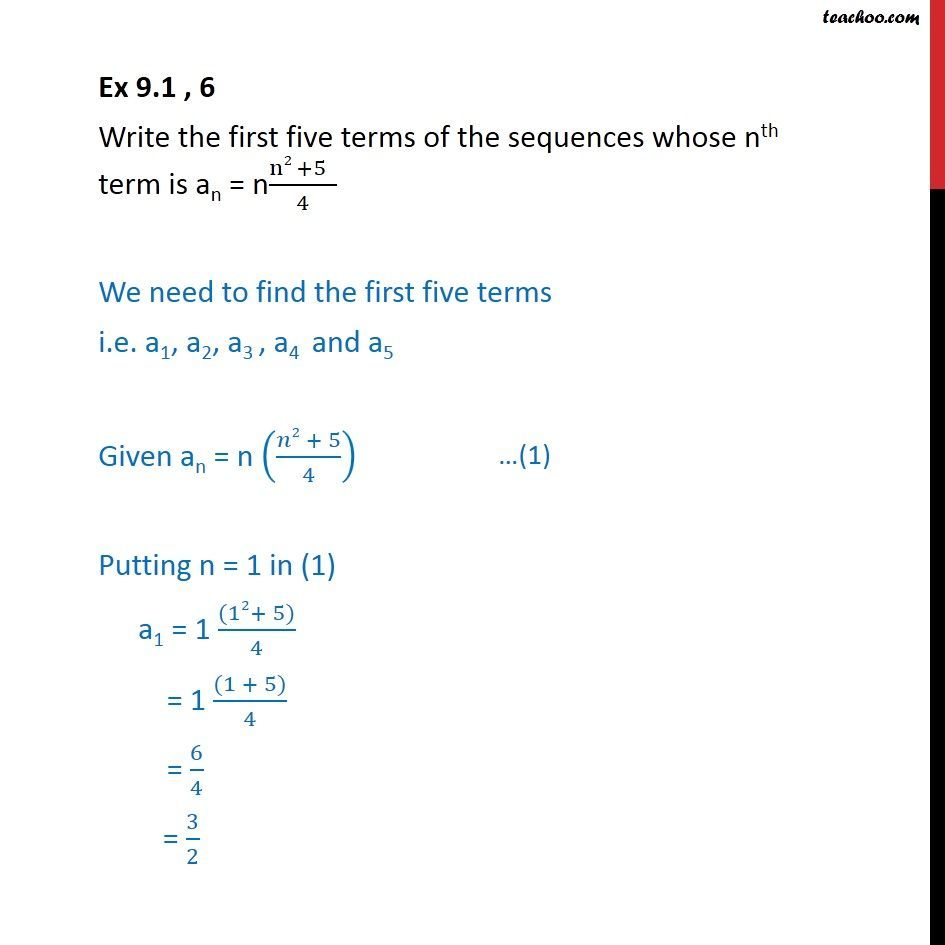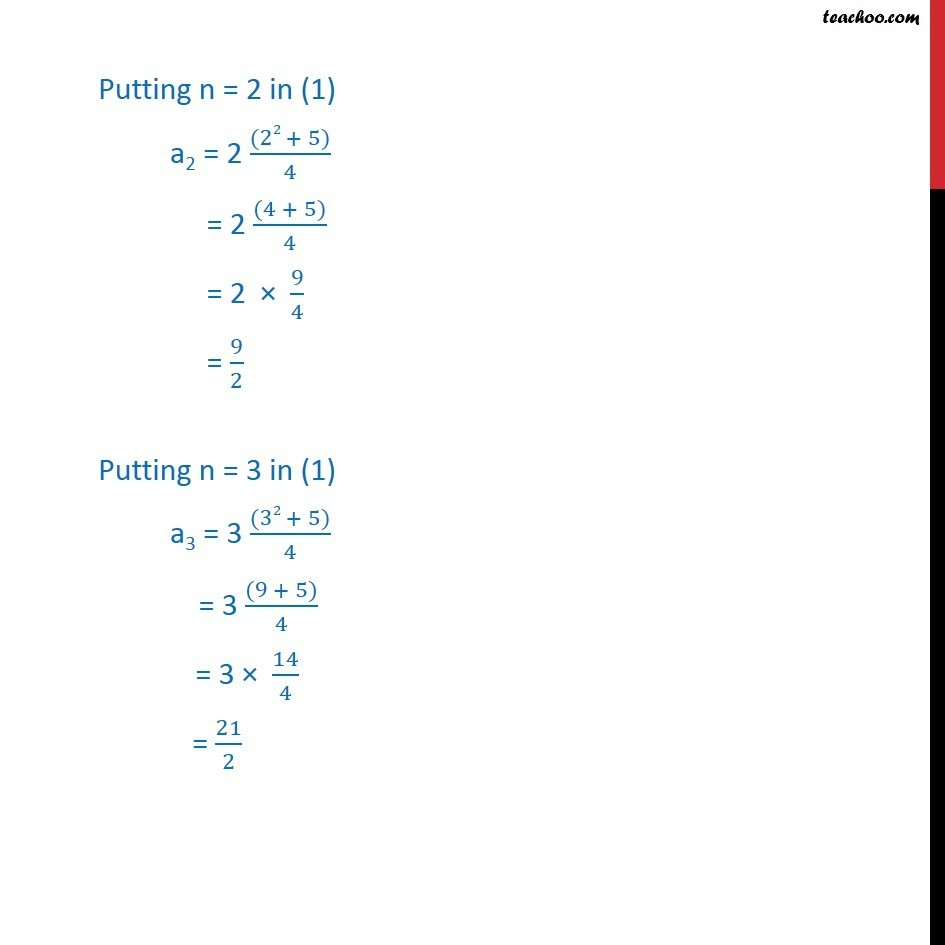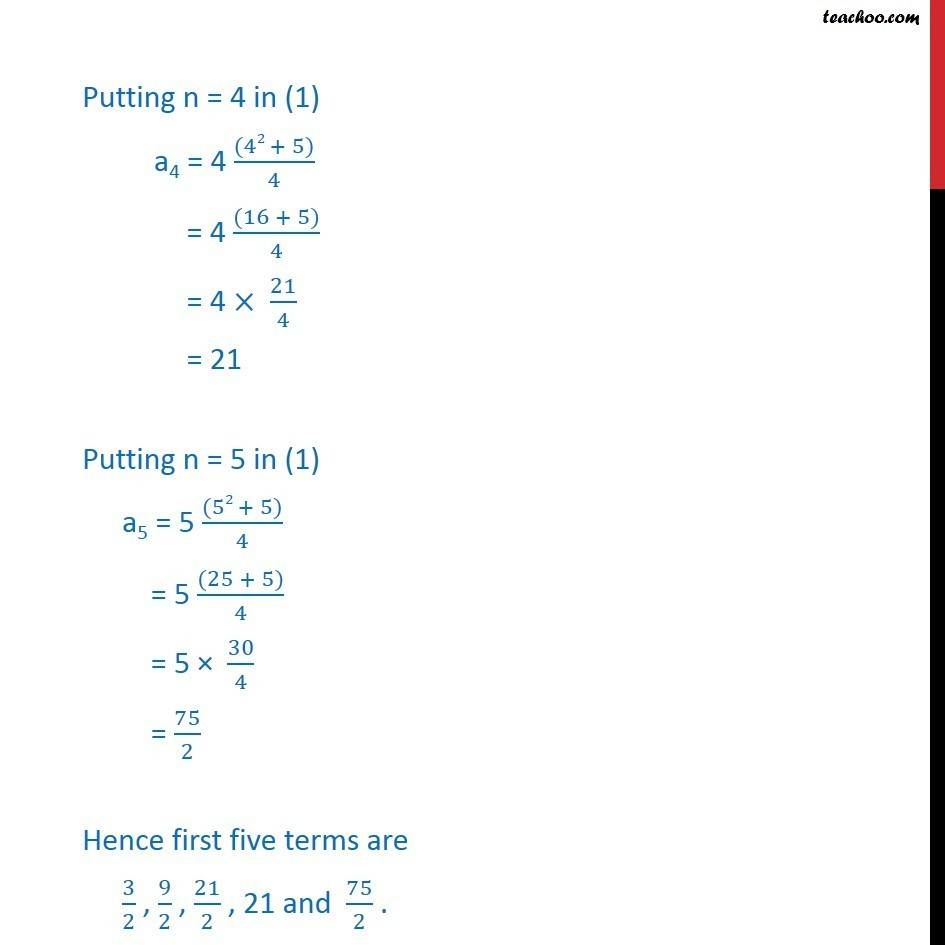Finding Sequences

Chapter 9 Class 11 Sequences and Series
Concept wiseIntroducing your new favourite teacher - Teachoo Black, at only ₹83 per month

### Transcript

Ex 9.1 , 6 Write the first five terms of the sequences whose nth term is an = n(n2 +5 )/4 We need to find the first five terms i.e. a1, a2, a3 , a4 and a5 Given an = n (( 2 + 5)/4) Putting n = 1 in (1) a1 = 1 ((12+ 5))/4 = 1 ((1 + 5))/4 = 6/4 = 3/2 Putting n = 2 in (1) a2 = 2 ((22 + 5))/4 = 2 ((4 + 5))/4 = 2 9/4 = 9/2 Putting n = 3 in (1) a3 = 3 ((32 + 5))/4 = 3 ((9 + 5))/4 = 3 14/4 = 21/2 Putting n = 4 in (1) a4 = 4 ((42 + 5))/4 = 4 ((16 + 5))/4 = 4 21/4 = 21 Putting n = 5 in (1) a5 = 5 ((52 + 5))/4 = 5 ((25 + 5))/4 = 5 30/4 = 75/2 Hence first five terms are 3/2 , 9/2 , 21/2 , 21 and 75/2 .